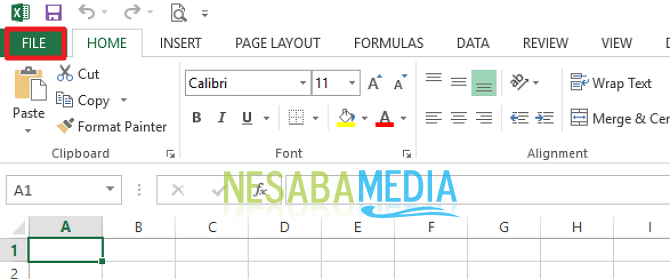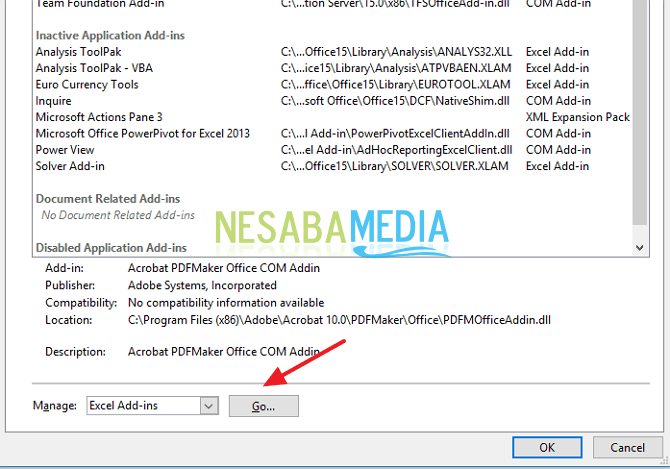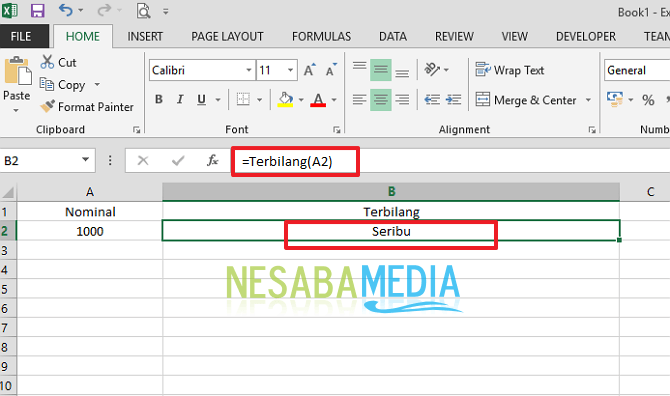Breaking News
Home / Computer / How to make numerical receipts in Microsoft Excel

How to make numerical receipts in Microsoft Excel

Microsoft Excel is a spreadsheet application developed by Microsoft for the Microsoft Windows operating system, Mac OS X, and iOS. There are so many features that can be used that are provided by Microsoft Excel such as graphics, calculations, tables and more. What's interesting about Microsoft Excel is the number of functions that can help complete work or tasks quickly.

Microsoft Excel's functions or functions are various, ranging from the simplest ones such as creating tables, processing data, calculating data, making diagrams making graphics and so on. Often we work using Microsoft Excel, we find data in the form of numbers such as the nominal price of Rp 120,000 must be changed to One Hundred Twenty Thousand Rupiah.

Generally this is used to make receipts in excel, such as financial reports and bookkeeping for companies or agency. Indeed, we can change it manually, but if the amount is large it will take a long time. But, it turns out we can change with the automatic method that is by using the function formula = spelled but to use this function we need several ways in Microsoft Excel. How is it done?

How to Make a Number Receipt Spelled in Microsoft Excel

Now, this time I will share about how to make a number receipt spelled out in Ms. Excel very easily. Just follow the steps – steps below, yes.

3. Then, please click File . As in the picture below.4. Then, please click "Options" . As in the picture below.6. Then, please click "Go" . As in the picture below.7. Then, please click "Browse" to enter the add-in file. As in the picture below.8. Then, please select the .xlam file that you downloaded earlier. Then, click Ok . As in the picture below.9. Then, please check the spelled box . Then, click Ok . As in the picture below.10. Then, you just use the formula. For example, you make a number on the A2 column 1945. Then, please make a formula in the column next to it = spelled (A2) then press enter . So, the results will be like in the picture below.In addition there are also some additional formulas that you can use

• to add the word to the back of it, examples of the formula = count (A2) & "rupiah"
• to make capital letters in every word, for example the formula = PROPER (spelled (A2) & "rupiah"
• to make all letters into uppercase letters, for example the formula [UPPER] (spelled (A2) & "rupiah"

Note:

• Function & is used to combine cell contents with formulas or with other cells
• PROPPER function is done to change the sentence format to the uppercase letter  UPPER  Function was used to change the sentence format to all capital letters

Now, how? The method is quite easy and very simple right? Now we can make receipts very easily. We do not need to write the nominal nominal In a manual way, we only need to make a formula and the results will automatically appear.

That is how to make a number receipt in Ms. Excel that you can easily try. If this article can help you, please share with your friends so that they also know how to make a number receipt in Ms. Excel easily. Hopefully this article can be useful for all of us. If there is a writing error, please forgive. Thank you 🙂